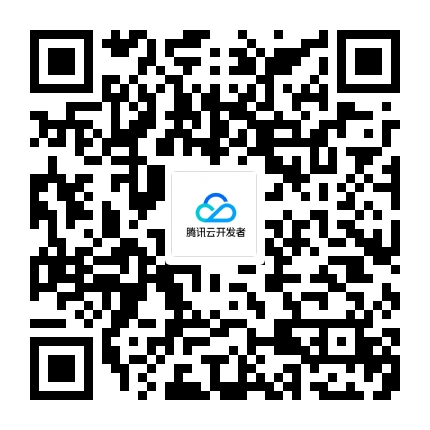TVP

# 2018年江苏省初中信息技术教师基本功大赛试题

G省将主办一次互联网产业大会，会议相关信息如下：

1. 呈现大会的基本信息。

2. 根据“任务一”资料包，生动呈现互联网发展情况。选择相关数据，用合适的方式进行表达，至少呈现两个以上不同类型的图表，并作相应的定性描述。

3.完成后，在“提交文件夹”文件夹中保存下列文件：宣传动画.fla,宣传动画.swf。

1. 在“画程”软件中绘制九九乘法表（输出样例如下图）的算法流程图。完成后，在“提交文件夹”文件夹中保存文件cf99.fld。

1*1=1

1*2=2 2*2=4

1*3=3 2*3=6 3*3=9

1*4=4 2*4=8 3*4=12 4*4=16

1*5=5 2*5=10 3*5=15 4*5=20 5*5=25

1*6=6 2*6=12 3*6=18 4*6=24 5*6=30 6*6=36

1*7=7 2*7=14 3*7=21 4*7=28 5*7=35 6*7=42 7*7=49

1*8=8 2*8=16 3*8=24 4*8=32 5*8=40 6*8=48 7*8=56 8*8=64

1*9=9 2*9=18 3*9=27 4*9=36 5*9=45 6*9=54 7*9=63 8*9=72 9*9=81

2. 请安装“任务四”资料包中的Python软件，根据第1小题在“画程”中生成的相应Python程序代码，在Python环境中，将“*”改成“×”，然后输出如下图的结果。完成后，在“提交文件夹”文件夹中保存文件cf99.py。

1.《江苏省义务教育信息技术课程纲要（2017年修订）》指出：学科核心素养是学科教育全面贯彻党的教育方针、match落实立德树人根本任务、实现素质教育的重要标match志，是学科育人价值的集中体现，是学生通过学科学习而逐步形成match的正确价值观念、必备品格和关键能力。请问信息技术学科核心素养包括哪些？请结合教学实例谈谈，你在教学中是如何引导学生运用计算机科学领域的思想方法，在问题解决过程中提升信息技术学科思维的。

2.“Alpha Go”是人工智能发展史上的重要事件之一。“Alpha Go”摒弃了“深蓝”采用的穷举法，它使用了深度学习的机器学习方式。请谈谈你对深度学习的理解。

3.对大数据的处理分析正成为新一代信息技术整合应用的结点。请说出大数据的主要特征。

4.智能产品需要借助传感器从外界获取信息。请说出传感器的工作原理，并列举智能手机中常用的传感器（至少5种）及其作用。

5.物联网的形成和发展得益于一次又一次的技术革新，其中离不开M2M技术。请问什么是M2M技术？物联网的架构由哪几层组成？

6.请说出视频编辑软件中轨道的作用，并列出视频编辑软件中常见的5种轨道。

7.在我们的生活中处处有排序，比如计算机系统中文件按时间排序、按类型排序等。请你写出两种排序算法的名称，并描述其中一种算法的排序过程（不需要写程序代码）。

8. 在数据的统计与分析中，雷达图是一种常见的图表类型。请说出雷达图的优点和缺点，其适用于什么类型的数据。

9.VR、AR和MR正在走进中小学课堂。请问它们分别是什么技术？你怎样看待它们进课堂？

10.区块链技术是比特币的底层技术。请问什么是区块链技术？一般来说，区块链系统由哪六层组成？match

• 发表于:
• 原文链接https://kuaibao.qq.com/s/20181029F19Q6100?refer=cp_1026
• 腾讯「腾讯云开发者社区」是腾讯内容开放平台帐号（企鹅号）传播渠道之一，根据《腾讯内容开放平台服务协议》转载发布内容。
• 如有侵权，请联系 cloudcommunity@tencent.com 删除。

2023-06-10

2023-06-10

2023-06-10

2023-06-10

2023-06-10

## 关注

10元无门槛代金券